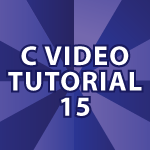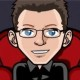# C Video Tutorial 15Welcome to part 15 of my C Video Tutorial! In this tutorial, I cover numerous topics.

First I go over how we can pass locations in memory between functions based on a request I received. Then I cover the Bitwise Operators AND, OR, Exclusive OR, and Shift Operators. We explore how signed numbers work and how the Ones Complement Operator can help. Then we look at Bit Masking and Binary Fractions. All of the code follows the video to help you learn.

Code From the Video

CTutorial15.c

```#include <stdio.h>
#include <stdlib.h>
#include <string.h>

char *convertBase(unsigned int numberToConvert, int base,
char *pConvertedNumber)
{

// All the potential numbers and letters in the converted number

char allValues[] = "0123456789abcdef";

if(base < 2 || base > 16){
printf("Enter a Number Between 2 and 16\n");
exit(1);
}

// Point to the last index in the character array
// Put \0 in that last index in the char array

pConvertedNumber = '\0';

do {

// Get the right index from allValues[] 0 through f

int index = numberToConvert % base;

// Decrement the pointer to the next index to the
// left in the char array

pConvertedNumber = pConvertedNumber - 1;

// Store the proper value in the char array for the
// final converted number

*pConvertedNumber = '0' + index;

// NEW *pConvertedNumber = allValues[index];

// Divide by the base to check for the next number

numberToConvert /= base;

} while(numberToConvert != 0);

return pConvertedNumber;

}

int main(void){

unsigned int numberSix = 6; // 110
unsigned int numberSeven = 7; // 111
unsigned int andSolution;

// Buffer that will hold 32 bits + a \0 at the end
char *pConvertedNumber;
pConvertedNumber = malloc(33 * sizeof(char));

printf("%s\n", convertBase(numberSix, 2, pConvertedNumber));

printf("%s\n", convertBase(10, 2, pConvertedNumber));

// Now I'll explore C's Bitwise Operators

// AND : If both are 1 & returns a 1 ------------
// 110 & 111 = 110

andSolution = numberSix & numberSeven;

printf("%s & ", convertBase(numberSix, 2, pConvertedNumber));
printf("%s = ", convertBase(numberSeven, 2, pConvertedNumber));
printf("%s\n\n", convertBase(andSolution, 2, pConvertedNumber));

// OR : If either are 1 | returns a 1 ------------
// 010 & 111 = 111

unsigned int orSolution = numberSix | numberSeven;

printf("%s | ", convertBase(numberSix, 2, pConvertedNumber));
printf("%s = ", convertBase(numberSeven, 2, pConvertedNumber));
printf("%s\n\n", convertBase(orSolution, 2, pConvertedNumber));

// EXCLUSIVE OR : 1 is returned only if one is 1 and the other 0
// 010 & 111 = 101

unsigned int exOrSolution = numberSix ^ numberSeven;

printf("%s ^ ", convertBase(numberSix, 2, pConvertedNumber));
printf("%s = ", convertBase(numberSeven, 2, pConvertedNumber));
printf("%s\n\n", convertBase(exOrSolution, 2, pConvertedNumber));

// SIGNED INTEGERS --------------------

// Signed integers can make things more complicated. A computer
// could use the highest order bit to represent the sign.
// So, 10000010 = -2 ( This isn't done though )
// This means the largest number is 127 and the smallest is -127
// 127 = 64 + 32 + 16 + 8 + 4 + 2 + 1

// Computers represent signed numbers using something called
// Two's Complement. To get -2 using TC you take 2 (00000010)
// and invert the numbers to (11111101) and then add 1
// or (11111110) = -2

// To convert -2 back to 2 you invert the numbers for -2 and add 1
// You know if a binary number is negative if the first digit is negative

// ONES COMPLEMENT OPERATOR: Flips all the bits
// 010 & 111 = 101

unsigned int onesCompSolution = ~numberSix;

printf("~%s = ", convertBase(numberSix, 2, pConvertedNumber));
printf("%s\n\n", convertBase(onesCompSolution, 2, pConvertedNumber));

printf("Negative of %d = %d\n\n", numberSix, (onesCompSolution + 1));

// SHIFT OPERATORS ----------------------

// BINARY LEFT SHIFT OPERATOR: Shifts the bits to the left
// 111 << 2 = 11100

unsigned int shiftLeftTwo = numberSix << 2;

printf("%s << 2 = ", convertBase(numberSix, 2, pConvertedNumber));
printf("%s = %d\n\n", convertBase(shiftLeftTwo, 2, pConvertedNumber),
shiftLeftTwo);

// BINARY RIGHT SHIFT OPERATOR: Shifts the bits to the right
// 111 >> 2 = 1

unsigned int shiftRightTwo = numberSix >> 2;

printf("%s >> 2 = ", convertBase(numberSix, 2, pConvertedNumber));
printf("%s = %d\n\n", convertBase(shiftRightTwo, 2, pConvertedNumber),
shiftRightTwo);

// BIT MASKING --------------------------- (FRAME 57)

// Bit Masking is used to select part of a series of bits.
// You can get a few bits by using the AND operator with
// 1s in the positions you are interested in and 0s otherwise.

unsigned int analyzeMyBits = 170; // 10101010

unsigned int theMask = 15; // 00001111

unsigned int last4Bits = analyzeMyBits & theMask;

// 		10101010
// AND	00001111
// 		00001010 = 10

printf("Last 4 Bits : %s\n", convertBase(last4Bits, 2, pConvertedNumber));

// To represent fractions in binary we do this:
// .625 * 2 = 1.25 ( Take the first digit 1 and save it )
// Move the .25 here and .25 * 2 = 0.50 ( Take the first digit 0
// and save it )
// .50 * 2 = 1.0 ( Take the first digit 1 and save it )
// When the fraction becomes 0 we are done and .625 is represented
// in binary as .101

// What happens with infinite fractions?
// .1 * 2 = 0.2 (Binary .0)
// .2 * 2 = 0.4 (Binary .00)
// .4 * 2 = 0.8 (Binary .000)
// .8 * 2 = 1.6 (Binary .0001)... This goes on forever, but the computer
// will only store so many

free(pConvertedNumber);
return 0;
}```

### 5 Responses to “C Video Tutorial 15”

1.lan says:

Good day. A quick question?
Wat kind of setup would you recommend
for studying device driver programming?

I was thinking a virtual machine, but would
a mistake in the code (possibly) render the
harware useless?

Is there a safe? way to experiment in driver programming
without having to fear the death of my (only) pc?

Thanks in advance and have a good day.

•Derek Banas says:

The only book I know of is Linux Device Drivers. I really want to get into electronics and cover everything starting with how an electron travels through a conductor. From there I want to work up to building real things. Even a computer. I’ll get there some day.

•lan says:

Hope we’re all blessed to live long enough
and in peace to see that day

cheers
p.s. Any advice on the setup: i mean on what kind of machine?

2.Aniket says:

Any chance you plan on tutorial on operating systems/ security/distributed systems

if any help could be provided on kernel programming/multithreading it would be greatly appreciated.

thanks and keep up the excellent work!! god bless you

•Derek Banas says:

Thank you very much and may God bless you as well. For now I want to focus on teaching Android. After I think I’ve done a good job of that I will move on to other topics.